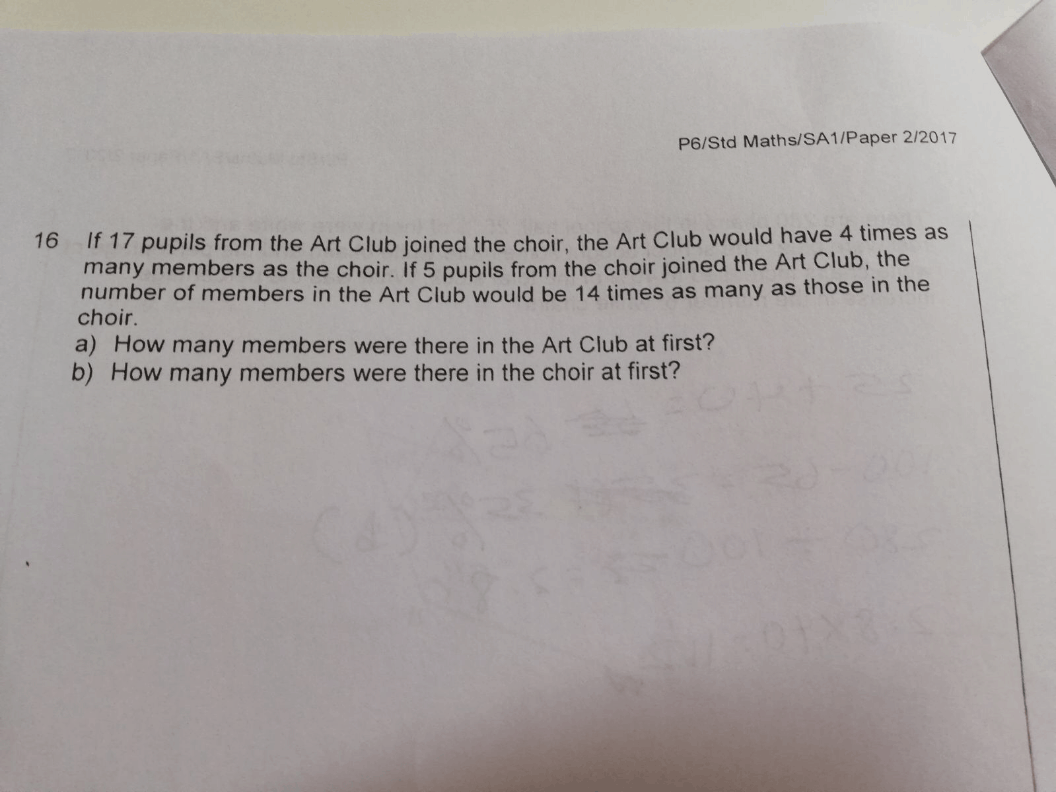# Questionappreciate some help

thanks

2 Answers

# Answer

(a)
If 17 pupils from the Art Club joined the choir,
Art Club : 4u
choir : 1u

At first —
Art Club : 4u + 17
choir : 1u – 17

If 5 pupils from the choir joined the Art Club,
Art Club : 14p
choir : 1p

At first —
Art Club : 14p – 5
choir : 1p + 5

4u + 17 ——-> 14p – 5
1u – 17 ——-> 1p + 5
5u ——-> 15p
1u ——-> 3p
3p – 17 ——-> 1p + 5
p ——-> (5 + 17)/(3 – 1) = 11
14p – 5 = 14 x 11 – 5 = 149

(b)1p + 5 = 11 + 5 = 16

Ans : (a) 149 members; (b) 16 members.

0 Replies 0 Likes ✔Accepted Answer

This is a After Before After question.

 After Transfer Before Taansfer After Ratio Art 4u + 17 4u + 17 + 5 4u + 22 14 Choir 1u – 17 1u – 17 – 5 1u – 22 1

(4u + 22) / 14   = (1u – 22) / 1

4u + 22 = 14u – 308

22 + 308 = 14u – 4u

10u = 330

1u = 330 ÷ 10 = 33

a)

4u + 17 = 4 × 33 + 17 = 149

b)

1u – 17 = 33 – 17 = 16

0 Replies 1 Like